# Excercise 7.2 Congruence-of-Triangles- NCERT Solutions Class 7

## Chapter 7 Ex.7.2 Question 1

Which congruence criterion do you use in the following?

 (a) Given: \begin{align}AC &= DF\\AB &= DE\\BC &= EF\end{align}                           So, $$ΔABC ≅ ΔDEF$$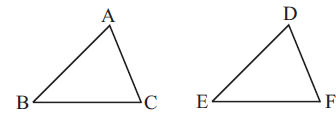(b) Given:  \begin{align} ZX &= RP\\RQ &= ZY\\∠PRQ &= ∠XZY \end{align} So, $$ΔPQR ≅ ΔXYZ$$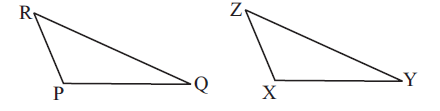(c) Given: \begin{align} ∠MLN &= ∠FGH\\∠NML &= ∠GFH\\ML &= FG \end{align} So, $$ΔLMN ≅ ΔGFH$$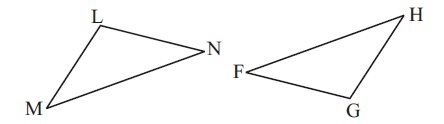(d) Given: \begin{align} EB &= DB\\AE &= BC\\∠A &= ∠C = 90^\circ \end{align} So, $$ΔABE ≅ ΔCDB$$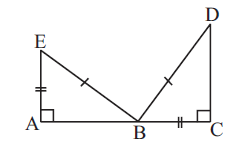### Solution

(a)

What is known?In $$ΔABC$$ and $$ΔDEF$$

\begin{align} AC&= DF\\AB &= DE\\BC &= EF \end{align}

What is the unknown?

Congruence criterion by which these two triangles are congruent.

Reasoning:

Three sides of a $$ΔABC$$ are equal to the corresponding three sides of the other $$ΔDEF$$. So, $$SSS$$ congruence criterion can be used.

Steps:

Given, $$AC$$ = $$DF$$, $$AB = DE$$ and $$BC = EF$$. The three sides of a $$ΔABC$$ are equal to the corresponding three sides of the other $$ΔDEF$$. So, by $$SSS$$ congruence criterion, the two triangles are congruent.

(b)

What is known?\begin{align} ZX &= RP\\RQ &= ZY\\∠PRQ &= ∠XZY \end{align}

What is unknown?

Congruence criterion by which the two triangles are congruent.

Reasoning:

The two sides and one angle of a $$ΔPRQ$$ are equal to the corresponding two sides and one angle of the other $$ΔXYZ$$. So, $$SSS$$ congruence criterion can be used.

Steps:

Given,$$ZX = RP$$, $$RQ = ZY$$, $$∠PRQ = ∠XZY$$. The two sides and one angle of a triangle $$ΔPRQ$$ are equal to the corresponding two sides and one angle of the other triangle $$ΔXYZ$$. So, by $$SAS$$ congruence criterion, the two triangles are congruent.

(c)

What is known?\begin{align} ∠MLN&= ∠FGH\\∠NML &= ∠GFH\\ML &= FG \end{align}

What is the unknown?

By which congruence criterion two triangles are congruent.

Reasoning:

The two angles and one side of a triangle $$ΔLMN$$ are equal to the corresponding two sides and one angle of the other triangle $$ΔGFH$$. So, by using congruency criterion based on two angels and one side $$(ASA)$$ of triangles can be used.

Steps:

Given, $$∠MLN = ∠FGH$$, $$∠NML = ∠GFH$$ and $$ML = FG$$. The two sides and one angle of triangle $$ΔLMN$$ are equal to the two sides and one angle of the other triangle $$ΔGFH$$. So, by $$ASA$$ congruence criterion, the two triangles are congruent.

(d)

What is known?In $$ΔABE$$ and $$ΔCDB$$

\begin{align} EB &= DB\\AE &= BC\\∠A& = ∠C = 90^\circ \end{align}

What is the unknown?

By which congruence criterion two triangles are congruent.

Reasoning:

In this case, the hypotenuse and one side of a right-angled triangle are respectively equal to the hypotenuse and one side of another right-angled triangle. congruency is based on of a right-angle, hypotenuse and one side $$(RHS)$$ criterion.

Steps:

Since, $$EB = DB$$, $$AE = BC$$, $$∠A = ∠C = 90^\circ$$. The hypotenuse and one side of a right-angled triangle $$ΔABE$$ are equal to the hypotenuse and one side of the other right-angled triangle $$ΔCDB.$$ So, by $$(RHS)$$ congruence criterion, the two triangles are congruent.

## Chapter 7 Ex.7.2 Question 2

You want to show that $$ΔART ≅ ΔPEN,$$

(a) If you have to use $$SSS$$ criterion, then you need to show

(i) $$AR$$ =

(ii) $$RT$$ =

(iii) $$AT$$ =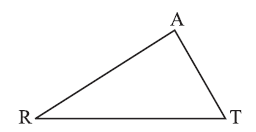(b) If it is given that $$∠T = ∠N$$ and you are to use $$SAS$$ criterion, you need to have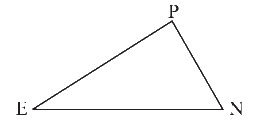(i) $$RT$$ =

(ii) $$PN$$ =

(c) If it is given that $$AT$$ = $$PN$$ and you are to use $$ASA$$ criterion, you need to have

(i) $$ZA$$

(ii) $$ZT$$

### Solution

Steps:

(a) If you have to use $$SSS$$ criterion, then you need to show

(i) $$AR$$ = $$PE$$

(ii) $$RT$$ = $$EN$$

(iii) $$AT$$ = $$PN$$

(b) If it is given that $$∠T = ∠N$$ and you are to use $$SAS$$ criterion, you need to have

(i) $$RT$$ = $$EN$$

(ii) $$PN$$ = $$AT$$

(c) If it is given that $$AT$$ = $$PN$$ and you are to use $$ASA$$ criterion to show that $$ΔART ≅ ΔPEN,$$ you need to have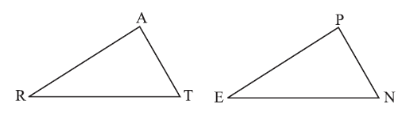(i) $$∠RAT = ∠EPN$$

(ii) $$∠RTA = ∠ENP$$

## Chapter 7 Ex.7.2 Question 3

You have to show that $$ΔAMP ≅ ΔAMQ$$. In the following proof, supply the missing reasons.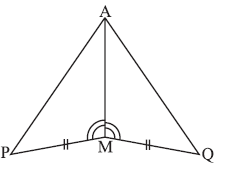Steps Reasons (i) $$PM = QM$$ (i) …. (ii) $$∠PMA = ∠QMA$$ (ii) …. (iii) $$AM = AM$$ (iii) …. (iv) $$ΔAMP ≅ ΔAMQ$$ (iv) ….

### Solution

Steps:

 Steps Reasons (i) $$PM = QM$$ (i) Given (ii) $$∠PMA = ∠QMA$$ (ii) Given (iii) $$AM = AM$$ (iii) Common (iv) $$ΔAMP ≅ ΔAMQ$$ (iv) $$SAS$$ congruence rule

## Chapter 7 Ex.7.2 Question 4

In $$ΔABC$$, $$∠A = 30^\circ$$, $$∠B = 40^\circ$$ and $$∠C = 110^\circ$$. In $$ΔPQR$$, $$∠P = 30^\circ$$, $$∠Q = 40^\circ$$ and $$∠R =110^\circ$$. A student says that $$ΔABC$$ $$≅$$ $$ΔPQR$$ by $$AAA$$ congruence criterion. Is he justified? Why or why not?

### Solution

What is known?

In $$ΔABC$$, $$∠A = 30^\circ$$, $$∠B = 40^\circ$$ and $$∠C = 110^\circ$$

In $$ΔPQR$$, $$∠P = 30^\circ$$, $$∠Q = 40^\circ$$ and $$∠R =110^\circ$$

What is the unknown?

Justification that $$ΔABC$$ $$≅$$ $$ΔPQR$$ by $$AAA$$ congruence criterion.

Reasoning:

In this question, it is given that the angle measure of all the angles of triangle $$ABC$$, $$∠A = 30^\circ$$, $$∠B = 40^\circ$$ and $$∠C = 110^\circ$$ is equal to the measure of all the angles of another triangle $$ΔPQR$$, $$∠P = 30^\circ$$, $$∠Q = 40^\circ$$ and $$∠R =110^\circ$$ and you can justify the congruence of $$ΔABC$$ and $$ΔPQR$$ by $$AAA$$ criterion or not. You can justify your answer by using the property based on $$AAA$$ congruence of two triangles. We know that there is no such thing as $$AAA$$ congruence of two triangles: Two triangles with equal corresponding angles need not to be congruent. In such a correspondence, one of them can be an enlarged copy of the other (They would be congruent only if they are exact copies of one another).

Steps:

No, $$AAA$$ property cannot justify $$ΔABC$$ $$≅$$ $$ΔPQR$$ because, this property represents that these two triangles have their respective angles of equal measures, but it gives no information about their sides. Two triangles with equal corresponding angles need not to be congruent. In such a correspondence, one of them can be an enlarged copy of the other. Therefore, $$AAA$$ does not prove that the two triangles $$ABC$$ and $$PQR$$ are congruent.

## Chapter 7 Ex.7.2 Question 5

In the figure, the two triangles are congruent. The corresponding parts are marked. We can write $$ΔRAT ≅$$?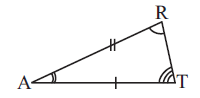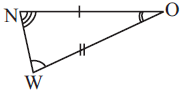### Solution

What is known?

The given two triangles are congruent and their corresponding parts are marked on the given figure.

$$\overline{NO}\text{ }=\text{ }\overline{AT}$$ , $$\overline{AR}\text{ }=\text{ }\overline{OW}$$

$$∠W = ∠R, ∠A = ∠O$$ and $$∠T = ∠N$$

What is the unknown?

Congruence of $$ΔRAT$$?

Reasoning:

This question is based on the concept of congruence of two triangle when two angles and one side (or two angles and one side) of the triangles are equal. If two angles and one side of a triangle are equal to the corresponding two angles and one side of another triangle then the two triangles can be congruent by $$ASA$$ Congruence criterion, by using this criterion you can find out the triangle congruent to $$RAT$$.

Steps:

From the figure, it can be observed that

\begin{align} \angle RAT &=\angle WON \\\angle ART & =\angle NOW \\ \overline{AR}\text{ } &=\text{ }\overline{OW}\end{align}

Therefore, $$ΔRAT ≅$$ WON (By $$ASA$$ congruence criterion)

## Chapter 7 Ex.7.2 Question 6

Complete the congruence statement: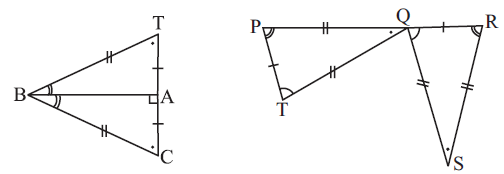(i) $$ΔBCA ≅ \,\,?$$

(i) $$ΔQRS ≅\,\,?$$

### Solution

What is known?What is the unknown?

Congruence of triangles.

(i) $$ΔBCA ≅ \,\,?$$

(ii)  $$ΔQRS ≅\,\,?$$

From figure, in the triangles $$BTA$$ and $$BCA,$$

$$B$$$$B,$$ $$A$$$$A$$ and $$T$$$$C$$

And in triangle $$QRS$$ and $$TPO,$$

$$P$$$$R,$$ $$T$$$$Q$$ and $$O$$$$S$$

Reasoning:

This question is based on the concept of congruence of triangles, if the corresponding parts of two triangles are equal then the two triangles are congruent to each other. In first triangle, corresponding parts of triangle $$BTA$$ are congruent to the triangle$$BCA$$ and the corresponding parts of triangle $$QRS$$ are congruent to triangle $$TPO.$$ Thus, by applying congruence based on sides of a triangle, two triangles can be proved congruent.

Steps:

In triangle $$BAT$$ and triangle $$BCA,$$ Corresponding parts are congruent.

$$B$$$$B,$$  $$A$$$$A$$ and $$T$$$$C.$$

So, by $$SSS$$ congruence rule, $$ΔBCA ≅ BTA$$

Similarly, in triangle $$QRS$$ and $$TPO,$$ corresponding parts are congruent. So, by $$SSS$$ congruence rule,

$$P$$$$R,$$ $$T ↔ Q$$ and $$O ↔ S$$

$$ΔQRS ≅ TPO$$

## Chapter 7 Ex.7.2 Question 7

In a squared sheet, draw two triangles of equal areas such that

(i) the triangles are congruent.

(ii) the triangles are not congruent.

What can you say about their perimeters?

### Solution

Steps:

Draw two triangles of equal areas,

(i)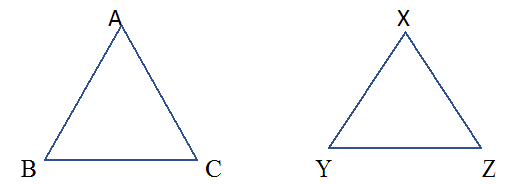Triangles $$ABC$$ and $$XYZ$$ have the same area and are congruent to each other. Also, perimeter of both the triangles will be equal.

Such that,  $$\overline{AB}\text{ }=\text{ }\overline{DE},\overline{BC}\text{ }=\text{ }\overline{EF}\;\rm{and}\;\overline{AC}\text{ }=\text{ }\overline{DF}$$
On adding, we get $$\overline{AB}+\overline{BC}+\overline{AC}=\overline{DE}+\overline{EF}+\overline{DF}$$
i.e, perimeter of both the triangles are equal.

(ii)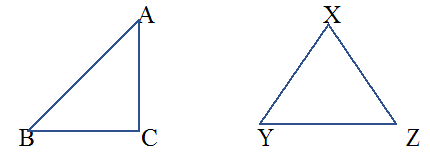Here, we have drawn two triangles $$ABC$$ and $$PQR$$ which are not congruent to each other.
Such that ,$$\overline{AB}\text{ }\ne \text{ }\overline{XY},\overline{BC}\text{ }\ne \text{ }\overline{YZ}\;\rm{and}\;\overline{AC}\text{ }\ne \text{ }\overline{XZ}$$
On adding, we get $$\overline{AB}+\overline{BC}+\overline{AC}=\overline{XY}+\overline{YZ}+\overline{XZ}$$
Also, the perimeter of both the triangles will not be the same.

## Chapter 7 Ex.7.2 Question 8

Draw a rough sketch of two triangles such that they have five pairs of congruent parts but still the triangles are not congruent.

### Solution

What is known?

Two triangles that have five pairs of congruent parts.

What is unknown?

Despite having five pairs of congruent parts, the triangles are not congruent

Steps:

In triangle $$PQR$$ and $$ABC$$

\begin{align}\overline{PQ}\text{ }&=\text{ }\overline{BC}\qquad\rm(Given)\\\overline{QR}\text{ }&=\text{ }\overline{AC}\qquad\rm(Given)\\\angle Q&=\angle B\qquad\rm(Given)\\\angle P &=\angle C\qquad\rm(Given)\\\angle R &=\angle A\qquad\rm(Given)\\\end{align}

All angles two sides are equal except one side. Hence triangle $$PQR$$ is not congruent to triangle $$ABC$$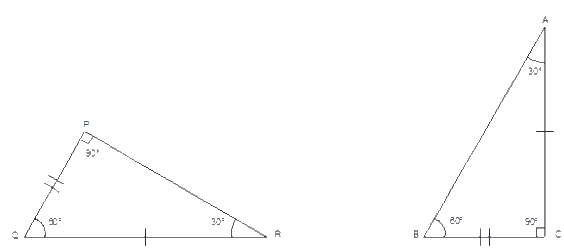## Chapter 7 Ex.7.2 Question 9

If $$ΔABC$$ and $$ΔPQR$$ are to be congruent, name one additional pair of corresponding parts. What criterion did you use?

### Solution

What is unknown?

Name one additional pair of corresponding parts and the criterion used.

Reasoning:

In this question, if two triangles $$ΔABC$$ and $$ΔPQR$$ are to be congruent, we must name one additional pair of corresponding part and the criterion used. For better understanding of this question, visualize it with the help of figure. In triangles $$ΔABC$$ and $$ΔPQR$$ it is given that, $$\angle B = 90^\circ$$ and $$\angle Q= 90^\circ\,, \angle C =\angle R.$$ Now, find out the side between these two angles that would be your one additional pair of corresponding part. Also, by reminding the criterion based on angle and the side of a right- angled triangle, you can find out the criterion used.

Steps:

In triangle $$ΔABC$$ and $$ΔPQR$$ are congruent.

$$\angle B = 90^\circ$$ and $$\angle Q= 90^\circ\,, \angle C =\angle R.$$

Then one additional pair is,

$$\overline {BC} = \overline{QR}$$

Therefore,

$$ΔABC ≅ PQR$$      (By $$ASA$$ congruence rule)

## Chapter 7 Ex.7.2 Question 10

Explain, why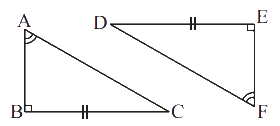$$ΔABC ≅ ΔFED$$.

### Solution

What is known?

Two right angles triangles $$ΔABC$$ and $$ΔFED$$ in which one side and one angle are equal.

What is unknown?

Why $$ΔABC ≅ ΔFED$$?

Reasoning:

This question is based on congruence of a right-angled triangle. In this question, one angle and a side of a right-angled triangle are equal to the corresponding one angle and a side of another right-angled triangle. Now, by using congruence based on right-angled triangle, you can find the reason why these two triangles are congruent.

Steps:

Given,

In triangles, $$ABC$$ and $$FED$$,

\begin{align}\angle \text{A}\,&=\ \angle \,\text{F}\\ \angle \text{B}\,&=\,\,\angle \text{E=9}{{\text{0}}^{\circ }}\\\rm{} BC &= ED\end{align}

Therefore, $$ΔABC ≅ ΔFED$$ (By $$RHS$$ congruence rule)

Instant doubt clearing with Cuemath Advanced Math Program